CATEGORIES:

Linear Operations on Vectors

Definition. The product of a vector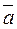and a real number l is the vectordefined by the following conditions:

(1)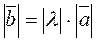;

(2) The vector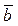is collinear to;

3. The vectorsandhave the same direction if λ>0 and opposite directions if λ<0. If λ=0, then the direction of the vectors is arbitrary.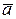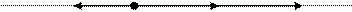3Property 1. For any numbers α and β and any vector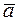,

α(β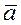)= (αβ)Definition. Suppose thatand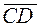are vectors and E is a point such that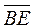=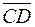. Then the vector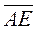is called the sum of the vectorsand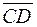and denoted by+Eε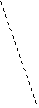ВA D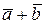C

Property 2. Addition of vectors is commutative; this means that, for any two vectors,=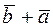.

Property 3. Addition of vectors is associative; this means that, for any vectors,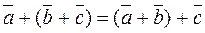.

Property 4. Addition is distributive with respect to multiplication by a number; i.e., for any vectorsand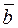and any number a,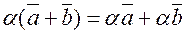.

Property 5. For any numbers a and b and any vector,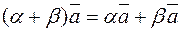.

Definition. Free vectors are vectors which can be translated, which means that they do not depend on the head but are determined by direction and length.

B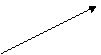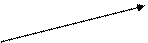B1 B

A A1 A

Consider vectors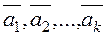, their sumis determined by one vector, whose head coincides with that of the first vector and the tail with that of the last vector.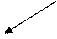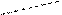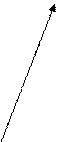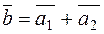,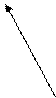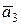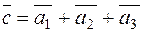.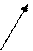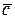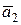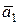Definition. The ort-vector of a vectoris the vector of unit length whose direction coincides with that of.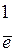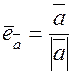is the ort-vector of.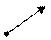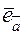Subtraction of vectors can be considered as the addition of two vectors, the second of which is taken with the sign –: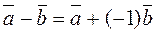.

Definition. The projection of a vector onto an axis is defined as the length of the interval whose endpoints are the projections of the endpoints of the vector onto this axis which is taken with the sign + if the angle between the vector and the axis is acute and with this sign – if this angle is obtuse:.

Date: 2015-01-02; view: 1141

 <== previous page | next page ==> The Intersection of a Plane and a straight Line | Decomposition of vectors.
doclecture.net - lectures - 2014-2022 year. Copyright infringement or personal data (0.004 sec.)